Скачать презентацию Significant Figure Rules How to round off when

b880c68dac74096b8b13641ab68cafc3.ppt

• Количество слайдов: 9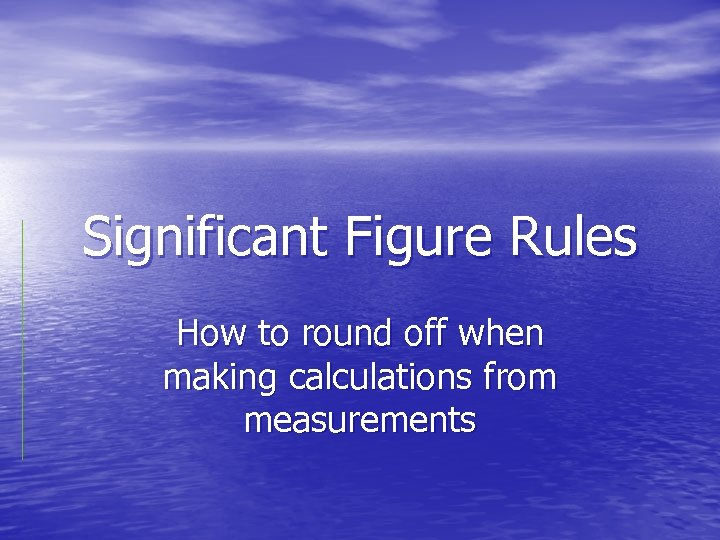Significant Figure Rules How to round off when making calculations from measurements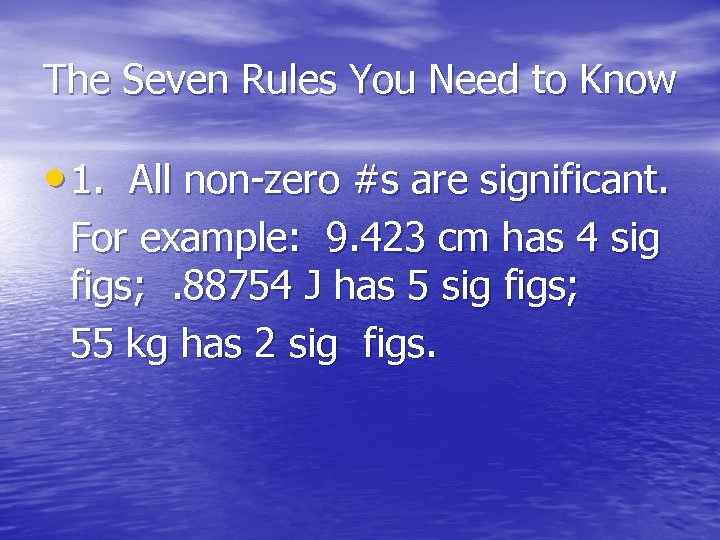The Seven Rules You Need to Know • 1. All non-zero #s are significant. For example: 9. 423 cm has 4 sig figs; . 88754 J has 5 sig figs; 55 kg has 2 sig figs.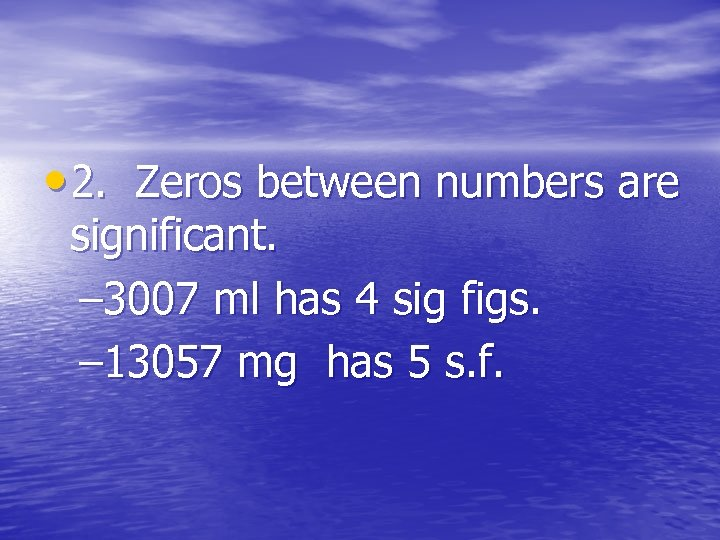• 2. Zeros between numbers are significant. – 3007 ml has 4 sig figs. – 13057 mg has 5 s. f.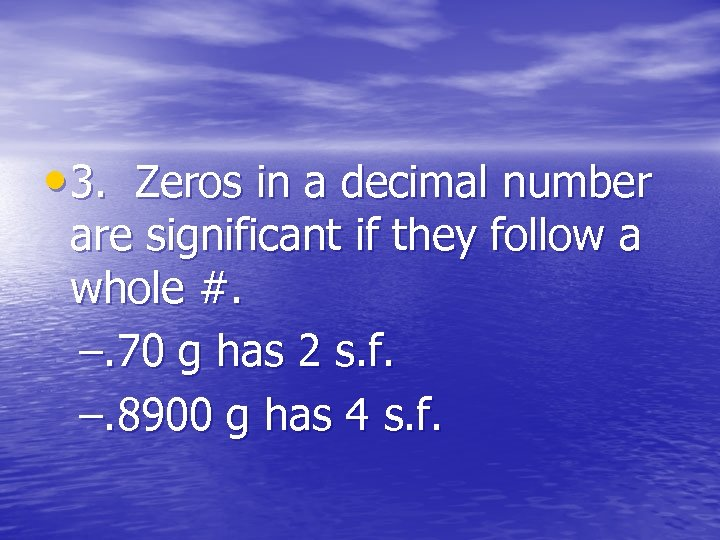• 3. Zeros in a decimal number are significant if they follow a whole #. –. 70 g has 2 s. f. –. 8900 g has 4 s. f.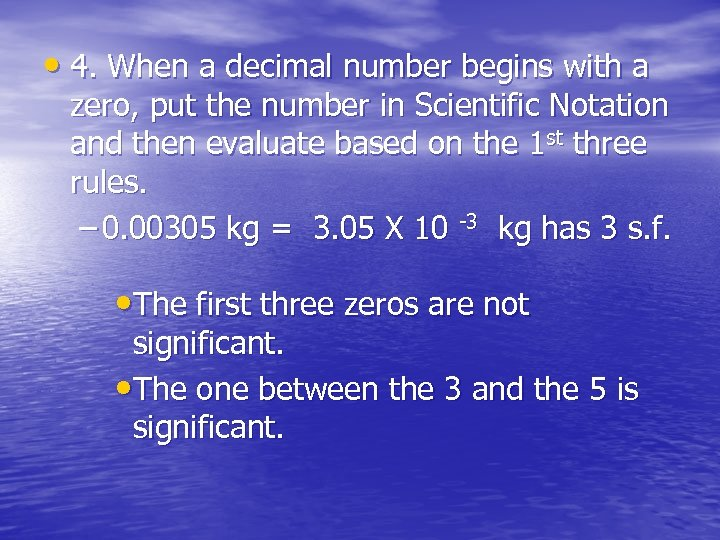• 4. When a decimal number begins with a zero, put the number in Scientific Notation and then evaluate based on the 1 st three rules. – 0. 00305 kg = 3. 05 X 10 -3 kg has 3 s. f. • The first three zeros are not significant. • The one between the 3 and the 5 is significant.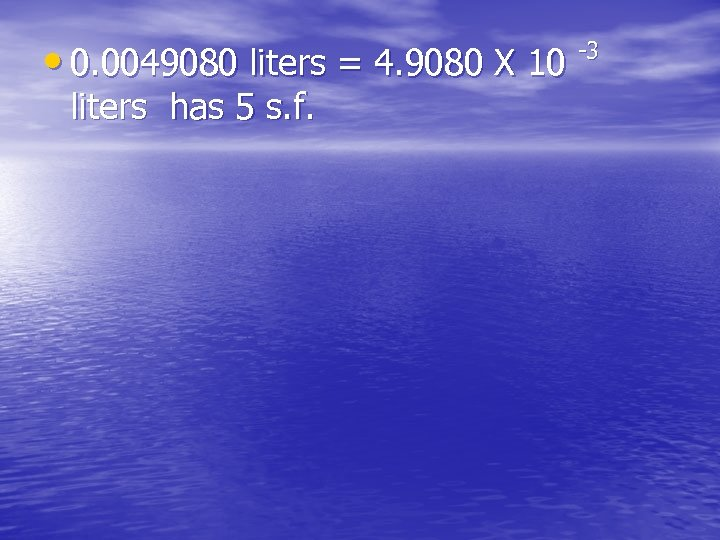• 0. 0049080 liters = 4. 9080 X 10 liters has 5 s. f. -3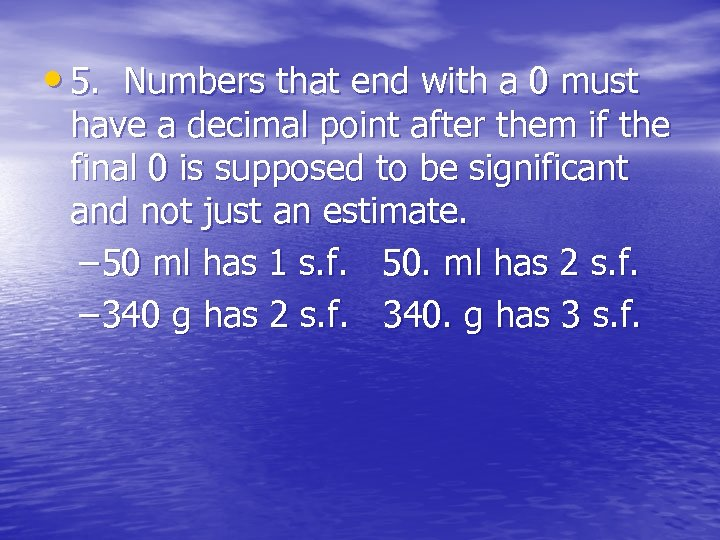• 5. Numbers that end with a 0 must have a decimal point after them if the final 0 is supposed to be significant and not just an estimate. – 50 ml has 1 s. f. 50. ml has 2 s. f. – 340 g has 2 s. f. 340. g has 3 s. f.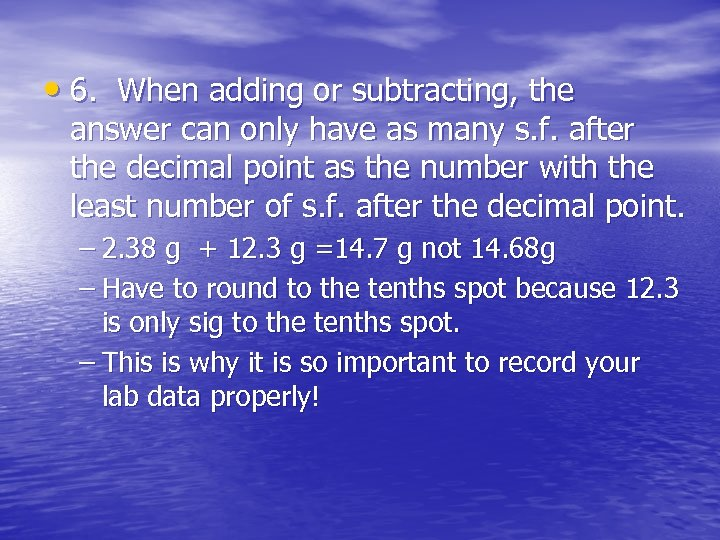• 6. When adding or subtracting, the answer can only have as many s. f. after the decimal point as the number with the least number of s. f. after the decimal point. – 2. 38 g + 12. 3 g =14. 7 g not 14. 68 g – Have to round to the tenths spot because 12. 3 is only sig to the tenths spot. – This is why it is so important to record your lab data properly!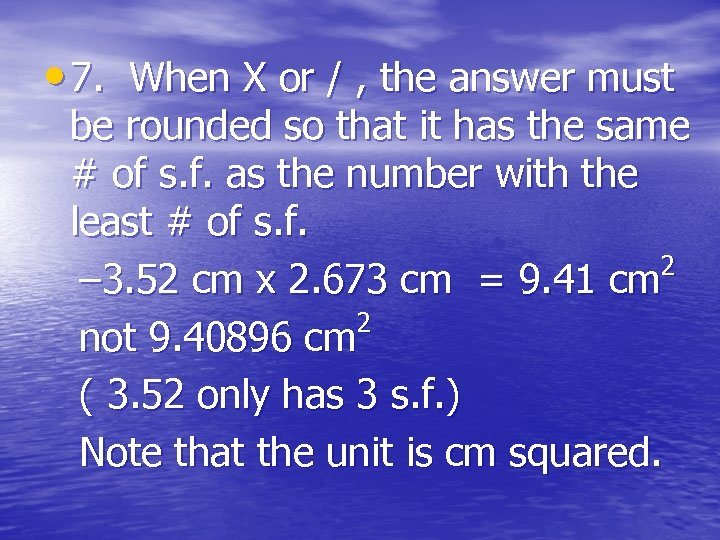• 7. When X or / , the answer must be rounded so that it has the same # of s. f. as the number with the least # of s. f. 2 – 3. 52 cm x 2. 673 cm = 9. 41 cm 2 not 9. 40896 cm ( 3. 52 only has 3 s. f. ) Note that the unit is cm squared.